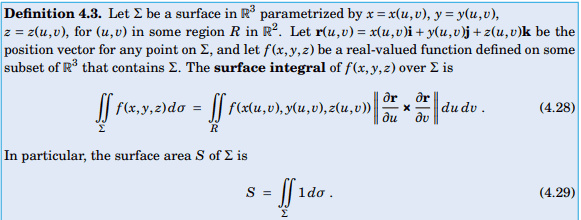# Evaluating $\iint\limits_{\sum} f \cdot d\sigma$ on Cube S

• MHB
• WMDhamnekar
In summary, we can evaluate the surface integral of $f(x,y,z) = xi + yj + zk$ over the boundary of the solid cube $S= \{(x,y,z) = 0\leq x,y,z \leq 1)\}$ without using the Divergence Theorem by parameterizing the six faces of the cube and using the formula for a surface integral of a vector field. The result is 3, which is the sum of the integrals over each individual face.f

#### WMDhamnekar

MHB
Without using the Divergence Theorem Evaluate the surface integral $\iint\limits_{\sum} f \cdot d\sigma$ of $f(x,y,z) = xi+ yj + zk , \sum:$ boundary of the solid cube S= $\{(x,y,z) = 0\leq x,y,z \leq 1)\}$

My attempt:
Here we have to use the following definition of surface integral.Note that there will be a different outward unit normal vector to each of the six faces of the cube.

Last edited:
Hi Dhamnekar Winod,

The formula you posted is for a real-valued function, but the function $f$ you provided is a vector field. You will need to use the formula for a surface integral of a vector field: $$\iint\limits_{R}f(x(u,v), y(u,v), z(u,v))\cdot \left(\frac{\partial\mathbf{r}}{\partial u}\times \frac{\partial\mathbf{r}}{\partial v} \right)du\,dv \qquad(*)$$ See the Wikipedia - Surface Integrals of Vector Fields.

After that, think about how to parameterize each of the six faces of $\Sigma$. For example, the "bottom" face can be parameterized as $x(u,v) = u$, $y(u,v) = v$, and $z(u,v) = 0$, where $0\leq u, v\leq 1$; i.e., $\mathbf{r}(u,v)= u\mathbf{i} + v\mathbf{j} + 0\mathbf{k}$ for $0\leq u, v\leq 1$. Using this parameterization, calculate the integral in $(*)$. Note: You will need to use the negative of the cross product $\frac{\partial\mathbf{r}}{\partial u}\times \frac{\partial\mathbf{r}}{\partial v}$ to ensure you get an outward pointing normal vector to the "bottom" of $\Sigma$. Then repeat the process for each of the other 5 faces of $\Sigma$.

Last edited:
This integral will be over the 6 faces of the cube. Do each separately. One face is z= 0 for x and y each from 0 to 1. On z= 0, the vector field zk= 0k so the integral is 0. That is also true on the faces x= 0 and y= 0. On z= 1 we integrate zk= 1k so we are integrating 1 for both x and y from 0 to 1. That is 1 times the area of the square, which is also 1, so the integral is 1. This is also true on the faces x= 1 and y= 1 so the entire surface integral is 3.

This integral will be over the 6 faces of the cube. Do each separately. One face is z= 0 for x and y each from 0 to 1. On z= 0, the vector field zk= 0k so the integral is 0. That is also true on the faces x= 0 and y= 0. On z= 1 we integrate zk= 1k so we are integrating 1 for both x and y from 0 to 1. That is 1 times the area of the square, which is also 1, so the integral is 1. This is also true on the faces x= 1 and y= 1 so the entire surface integral is 3.
f(x,y,z) = xi + yj + zk , $\vert \frac{dr}{du} \times \frac{dr}{dv} \vert =1$ So, $\iint\limits_{\sum} = f\cdot d\sigma =\displaystyle\int_0^1 \displaystyle\int_0^{1-x} (x+y+1-(x+y))\cdot (1)dydx=\frac12$

This surface integral is for one face of the cube. So entire surface integral will be $\frac12 \times 6 =3$

Last edited: